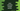# Java program to calculate student's grades in 2 different ways## Java program to calculate student’s grades:

In this post, we will learn different ways to calculate the grade of a student in Java. We will write one Java program that will ask the user to enter the marks of different subjects for a student. It will then calculate the average of the marks and based on the average value, it will print the grade.

We will use predefined grade mapping i.e. the grades will be defined before the average is calculated. The number of subjects might be different for different students. So, this program will also take the number of subjects as another input.

The program will use the following average marks and grade mapping:

>=80 A
>=60 and <80 B
>=30 and <60 C
<30 D

To find the average marks, we will have to add the marks and divide the total sum by the number of subjects.

### Example 1: Java program to calculate student grade by using a for loop:

In this program, we will use a for loop to calculate the grade. Below is the complete program:

``````import java.util.Scanner;

public class Main {

public static void main(String[] args) {
float totalSubjects, totalMarks = 0, averageMarks;
Scanner scanner = new Scanner(System.in);

System.out.println("Enter the total number of subjects: ");
totalSubjects = scanner.nextFloat();

for (int i = 1; i <= totalSubjects; i++) {
System.out.println("Enter the marks for subject " + i + ": ");
totalMarks += scanner.nextFloat();
}

averageMarks = totalMarks / totalSubjects;

if (averageMarks >= 80) {
System.out.println("Average Marks: " + averageMarks + ", Grade A");
} else if (averageMarks >= 60) {
System.out.println("Average Marks: " + averageMarks + ", Grade B");
} else if (averageMarks >= 30) {
System.out.println("Average Marks: " + averageMarks + ", Grade C");
} else {
System.out.println("Average Marks: " + averageMarks + ", Grade D");
}
}
}``````

Here,

• We created three floating point variables, totalSubjects is used to keep the total number of subjects, totalMarks is used to keep the total marks of all subjects and averageMarks is used to keep the average marks of all subjects. Also, one Scanner object is created to read the user input values.
• Once the user enters the total number of subjects, it reads that value and assigns it to the totalSubjects variable.
• The for loop is used to read the marks of the subjects one by one. It asks the user to enter the marks and it adds that value to the totalMarks variable.
• The average mark is calculated by dividing the total marks by the total number of subjects.
• The final if, else-if, else statements are used to find the grade. It compares the average mark and prints out the grade and average marks calculated.

This program will give the output as below:

``````Enter the total number of subjects:
3
Enter the marks for subject 1:
92
Enter the marks for subject 2:
85
Enter the marks for subject 3:
89

Enter the total number of subjects:
3
Enter the marks for subject 1:
87
Enter the marks for subject 2:
88
Enter the marks for subject 3:
35

This program will work with any number of subjects.

### Example 2: Java program to calculate student’s grade by using separate functions:

We can also use a separate function to calculate the student’s grade with separate functions. We can create one function to read the marks and another function to print the grades. Let’s write down the program:

``````import java.util.Scanner;

public class Main {

private static float getAverage() {
float totalSubjects, totalMarks = 0, averageMarks;
Scanner scanner = new Scanner(System.in);

System.out.println("Enter the total number of subjects: ");
totalSubjects = scanner.nextFloat();

for (int i = 1; i <= totalSubjects; i++) {
System.out.println("Enter the marks for subject " + i + ": ");
totalMarks += scanner.nextFloat();
}

}

private static void printGrade(float averageMarks) {
if (averageMarks >= 80) {
System.out.println("Average Marks: " + averageMarks + ", Grade A");
} else if (averageMarks >= 60) {
System.out.println("Average Marks: " + averageMarks + ", Grade B");
} else if (averageMarks >= 30) {
System.out.println("Average Marks: " + averageMarks + ", Grade C");
} else {
System.out.println("Average Marks: " + averageMarks + ", Grade D");
}
}

public static void main(String[] args) {